Reach Us+44 1704 335730
Pseudo-gradient Based Particle Swarm Optimization with Constriction Factor for Multi Objective Optimal Power Flow | OMICS International
Global Journal of Technology and Optimization
All submissions of the EM system will be redirected to Online Manuscript Submission System. Authors are requested to submit articles directly to Online Manuscript Submission System of respective journal.

# Pseudo-gradient Based Particle Swarm Optimization with Constriction Factor for Multi Objective Optimal Power Flow

Dieu Ngoc Vo1*, Tung The Tran1 and Tuan Trong Nguyen2

1Department of Power Systems, Ho Chi Minh City University of Technology, VNU-HMC, Ho Chi Minh City, Vietnam

2Southern Electrical Testing Company, Southern Power Company, Ho Chi Minh City, Vietnam

Corresponding Author:
Dieu Ngoc Vo
Department of Power Systems
Ho Chi Minh City University
of Technology VNU-HMC
Ho Chi Minh City, Vietnam
Tel: +84 8 3865 3823
E-mail: [email protected]

Received Date: March 20, 2015 Accepted Date: April 07, 2015 Published Date: April 30, 2015

Citation: Vo DN, Tran TT, Nguyen TT (2015) Pseudo-gradient Based Particle Swarm Optimization with Constriction Factor for Multi Objective Optimal Power Flow. Global J Technol Optim 6:181. doi:10.4172/2229-8711.1000181

Copyright: © 2015 Vo DN, et al. This is an open-access article distributed under the terms of the Creative Commons Attribution License, which permits unrestricted use, distribution, and reproduction in any medium, provided the original author and source are credited.

Visit for more related articles at Global Journal of Technology and Optimization

#### Abstract

This paper proposes a pseudo-gradient based particle swarm optimization with constriction factor (PG-PSOCF) method for solving multiobjective optimal power flow (MOOPF) problem. The proposed PG-PSOCF is the conventional particle swarm optimization based on constriction factor based on pseudo gradient to enhance its search ability for optimization problems. The proposed method is to deal with the MOOPF problem by minimizing the total cost and emission from generators while satisfying various constraints of real and reactive power balance, real and reactive power limits, bus voltage limits, shunt capacitor limits and transmission limits. Test results on the IEEE 30-bus system have indicated that the proposed method is more efficient than many other methods in the literature. Therefore, the proposed PG-PSOCF can be an effectively alternative method for solving the MOOPF problem.

#### Keywords

Constriction factor; Multiobjective optimal power flow; Particle swarm optimization; Pseudo gradient

#### Introduction

The objective of the optimal power flow (OPF) problem is to optimally determine the combination of control variables in power systems such as real power outputs of generators, voltage magnitude at generation buses, position of transformer tap changers, and reactive power outputs of shunt capacitors so that the total cost of thermal generators is minimized [1,2]. In fact, the OPF problem is a nonlinear and large-scale problem since it deals with several variables and nonlinear objective and constraints. Therefore, the OPF problem is always a challenge for solution methods, especially for those with non-differentiable objective functions which cannot be solved by conventional methods. Moreover, the power generation is also a source to release sulphur oxides (SOx), nitrogen oxides (NOx) and carbon dioxides (CO2) into the atmosphere. The US Clean Air Act amendments of 1990  has forced the utilities to adjust their power generation strategies to guarantee a minimum pollution level. Therefore, the OPF problem should also include the emission in its objective to form a multiobjective OPF (MOOPF) problem. The MOOPF problem is to simultaneously minimize total cost and emission of thermal generators while satisfying all unit and system constraints .

There have been several conventional methods proposed for solving the OPF problems such as gradient-based method , linear programming (LP) , non-linear programming (NLP) , quadratic programming (QP) , Newton-based methods , semidefinite programming , and interior point method (IPM) . In general, these conventional methods can easily find the optimal solution for a small-scale optimization problem in a very short time. However, the main disadvantage of them is that they suffer difficulty when dealing with non-convex optimization problems with non-differentiable objective functions. Moreover, they are also very difficult for dealing with large-scale problems due to large search space, leading time consuming or no convergence. The meta-heuristic search methods have recently developed shown that they are appropriate for dealing with complicated optimization problems, especially for those with non-differentiable objective functions. Several meta-heuristic search methods have been also widely applied for solving the OPF problem such as genetic algorithm (GA) , simulated annealing (SA) , tabu search (TS) , evolutionary programming (EP) [14,15], differential evolution (DE) , improved particle swarm optimisation (IPSO) [17,18], and modified shuffle frog leaping algorithm (MSFLA) . These meta-heuristic search algorithms can overcome the main drawback suffered by the conventional methods; that means they can deal with the problems which do not require objective functions to be differentiable. However, these meta-heuristic search methods may suffer near optimum solution and the solution quality may not high when dealing with large-scale and complex problems. That is the obtained solutions obtained by the methods may be local optima with long computational time. Therefore, the hybrid methods have also developed to overcome the drawback from the single meta-heuristic methods such as hybrid TS/SA , hybrid GA-IPM , hybrid differential evolution , hybrid of fuzzy and PSO , and geneticbased fuzzy mathematical programming technique . The aim of the hybrid methods is to utilize the advantages from each element method to obtain the better optimal solution. Although the hybrid methods can obtain better solution quality than the single methods, they may be suffered slower computational time than the single methods due to combination of many operations. Moreover, the hybrid systems are also usually more complex than the element methods.

In this paper, a pseudo-gradient based particle swarm optimization with constriction factor (PG-PSOCF) method is proposed for solving the MOOPF problem. The proposed PG-PSOCF is the conventional particle swarm optimization based on constriction factor based on pseudo gradient to enhance its search ability for optimization problems. The proposed method is to deal with the MOOPF problem by minimizing the total cost and emission from generators while satisfying various constraints of real and reactive power balance, real and reactive power limits, bus voltage limits, shunt capacitor limits and transmission limits. Test results on the IEEE 30-bus system have indicated that the proposed method is more efficient than many other methods in the literature.

The remaining organization of this paper is follows. Section 2 addresses the formulation of MOOPF problem. A PG-PSOCF implementation for the problem is described in Section 3. Numerical results are presented in Section 4. Finally, the conclusion is given.

#### The MOOPF Problem Formulation

The objective of the MOOPF problem is to simultaneously minimize the both total cost and emission while satisfying several equality and inequality constraints. Mathematically, the problem is formulated as follows:

Minimize F1(u,x), F2(u,x) (1)

subject to g(u,x) = 0 (2)

h(u,x) ≤ 0 (3)

where F1(u,x) and F2(u,x) are the objective functions representing total cost and emission, respectively; g(u,x) represents the equality constraints representing power balance at buses; h(u,x) represents the inequality constraints representing upper and lower limits of real power outputs, reactive power outputs, bus voltages, transformer tap changers, shunt capacitors, and power flow in transmission lines; u is the vector of the control variables including active power outputs of generators, magnitudes of generation bus voltage, transformers taps, and shunt capacitors; and x represents state variables including reactive power output, magnitudes of load bus voltage , bus voltage angles, and power flow in transmission lines.

The fuel cost function (\$/h) of generators in form of quadratic function is represented by: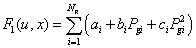(4)

where Ng is the number of generators including the slack bus; Pgi is the active power output of generator at bus i; ai, bi and ci are the cost coefficients of generator i.

The total emission (ton/h) from generators is represented by: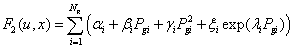(5)

where αi, βi, γi, ξi, and λi are emission coefficients of generator i.

The equality and inequality constraints of the problem represented mathematical model as follows:

a) Real and reactive power flow equations at each bus: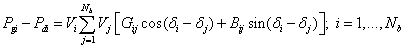(6)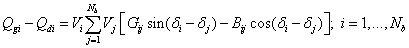(7)

b) Voltage and reactive power limits at generation buses: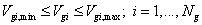(8)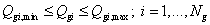(9)

c) Capacity limits for switchable shunt capacitor banks: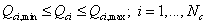(10)

d) Transformer tap settings constraint: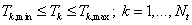(11)

e) Security constraints for voltages at load buses and transmission lines: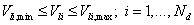(12)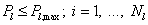(13)

where Qgi is reactive power outputs of generating unit i; Pdi and Qdi are real and reactive load demand at bus i, respectively; Nb is the number of buses; Vi and θi are voltage magnitude and angle at bus i, respectively; Gij and Bij are transfer conductance and susceptance between bus i and bus j, respectively; Vgi is voltage at generation bus i; Qci is reactive power compensation source at bus i; Nc is the number of shunt capacitors; Tk is tap-setting of transformer branch k; Nt is the number of transformers; Vli is voltage magnitude at load bus i; Nd is the number of load buses; Pl is power flow in transmission line l connecting between bus i and bus j; and Nl is the number of transmission lines.

For the MOOPF problem formulation, the vector of control variables u is represented by: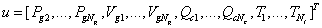(14)

where bus 1 is selected as the reference bus and the vector of the state variables x represented by:(15)

Pseudo-Gradient Based Particle Swarm Optimization with Constriction Factor

Particle swarm optimization with constriction factor

The conventional PSO was developed in 1995 by Kennedy and Eberhart . So far, this method has become one of the most popular meta-heuristic search methods implemented in the optimization problems of many fields due to its simplicity in application and efficiency in finding near optimum solution. The principle of PSO for searching the optimal solution for a problem is based on a population of particles which moves in the search space of the problem. The movement of the particles is determined via its location and velocity. During the movement, the position of particles will be updated according to the change of their velocity.

For application of PSO to find the optimal solution of an n-dimension problem, a population of NP particles will be used where the position and velocity vectors of particle d are represented by xd = [x1d, x2d, …, xnd] and vd = [v1d, v2d, …, vnd], respectively, where d = 1,…, NP. At each step, the best position of each particle represented by pbestd = [p1d, p2d, …, pnd] (d = 1,…, Np) based on the valuation of the fitness function and the best particle in the population represented by gbest will be stored for the next step. The velocity of each particle in the next iteration (k+1) for fitness function evaluation is calculated by: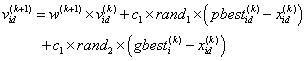(16)

where the constants c1 and c2 are cognitive and social parameters, respectively and rand1 and rand2 are random values in [0, 1].

The position of the corresponding particle is updated as follows: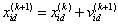(17)

Generally, the solution quality of the PSO method for optimization problems is sensitive to the calculation of the velocity of particles. Therefore, there have been several improvements on the calculation of velocity of particles to enhance its search ability and solution quality. Clerc and Kennedy have proposed an improvement of velocity calculation for particles with added constriction factor  which is to insure the stable convergence of the PSO algorithm. The modified velocity of particles with constriction factor C is calculated as follows: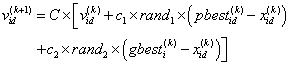(18)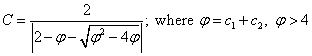(19)

In this improvement, the factor Ï� has an impact on the convergence characteristic of the method and must be greater than 4.0 for convergence stability. In the contrary, if the value of Ï� is high, the constriction C will be small, leading diversification and slower response. Therefore, the best typical value of Ï� suggested by Lim, Montakhab and Nouri  is 4.1 (i.e. c1=c2=2.05).

The pseudo-gradient is usually used for determining the maximum rate of change direction of non-differentiable functions where the conventional gradient is not applicable. Therefore, it is appropriate for using in population based search methods to enhance their search ability. This concept has been used in population based methods such as genetic algorithm  and evolutionary programming .

For a non-differentiable objective function f(x) where x=[x1, x2, …, xn] in a n-dimension optimization problem, a pseudo-gradient gp(x) for the objective function at a certain point xk=[xk1, xk2, …, xkn] in the search space of the problem moving to another one xl is defined for the two cases as follows :

i) f(x1) < f(xk): the direction from point xk to point x1 is defined as the positive direction. The pseudo-gradient at point x1 is determined by: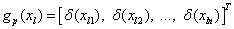(20)

where δ(xli) is the direction indicator for element xi moving from point k to point l defined by: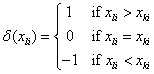(21)

ii) f(xl) ≥ f(xk): the direction from point xk to point xl is defined as the negative direction. The pseudo-gradient at point xl is determined by: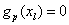(22)

As shown in the definition, if the value of the pseudo-gradient gp(xl)≠0, a better solution for the problem could be found in the next step based on the direction of the pseudo-gradient gp(xl) at point l. On the contrary, the search direction at this point may not appropriate due to no improvement can be found for the problem based on this direction.

Pseudo-gradient based particle swarm optimization

In this paper, the proposed PG-PSOCF is the PSO with constriction factor guided by pseudo-gradient to form a new improved PSO method.

For implementation of the pseudo-gradient in PSOCF, the two considered points for calculation of the pseudo-gradient include the particle’s position at iterations k and k+1 those are x(k) and x(k+1), respectively. Therefore, the updated position for particles in (17) can be rewritten as: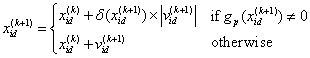(23)

As observed in (23), if the value of the pseudo-gradient is non-zero, the particle is moving on the right direction to the optimal solution in the search space of the problem with the enhanced velocity. Otherwise, the particle’s position is normally updated as in (17). With the implementation of the pseudo-gradient in PSOCF, the new improved PG-PSOCF can be more effective than the conventional PSO in solving optimization problems due to the enhanced search ability.

Implementation of PG-PSOCF for the MOOPF

For implementation of the proposed PG-PSOCF to the MOOPF problem, each particle position representing a vector of control variables is defined as follows:(24)

The upper and lower boundaries of the position of particles xd are also the upper and lower limits of the variables contained in the vector. The upper and lower limits for the velocity of each particle are determined based on their lower and upper bounds of position: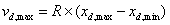(25)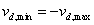(26)

where R is the limit factor for velocity of particles.

The positions and velocities of particles are randomly initialized within their limits as follows: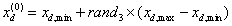(27)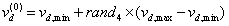(28)

where rand3 and rand4 are random values in [0, 1].

During the iterative process, the positions and velocities of particles are always adjusted satisfying their limits after each iteration as follows: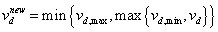(29)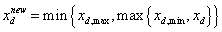(30)

The fitness function of the problem is defined based on the problem objective functions and the dependent variables including real power output at reference bus, reactive power outputs at generation buses, load bus voltages, and power flow in transmission lines. The fitness function of the problem is represented as follows: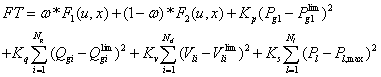(31)

where ω is the weight factor for objectives; Kp, Kq, Kv, and Ks are penalty factors for real power at reference bus, reactive power at generation buses, load bus voltages, and power flow in transmission lines, respectively.

The limits of the state variables in (31) are determined based on their calculated values as follows: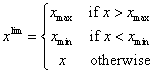(32)

where x and xlim respectively represent the calculated values and limits of Pg1, Qgi, Vli, or Pl,max

The overall procedure of the proposed PG-PSOCF for solving the OPF problem is addressed as follows:

Step 1: Select the controlling parameters for PG-PSOCF including number of particles NP, maximum number of iterations Itmax, cognitive and social acceleration factors c1 and c2, limit factor for maximum velocity R, and penalty factors for constraints in fitness function (31). Set the pseudo-gradient to zeros.

Step 2: Initialize the initial position xid and velocity vid of Np particles within in their limits.

Step 3: For each particle, calculate value of the state variables based on the power flow solution using Newton-Raphson and evaluate the fitness function Fpbestd in (31). Determine the best particle with the lowest value of fitness function Fgbest=min(Fpbestd, d=1,…, NP).

Step 4: Set the best particle’s position of each particle pbestid to xid, d=1,…, NP and the best particle in the population gbesti to the position of the particle corresponding to Fpbestd in Step 3. Set iteration counter k=1.

Step 5: Calculate new velocity v(k) id using (18) and update position x(k) id using (23) for each particle. Note that the obtained position and velocity of particles should be satisfied their lower and upper bounds given by (29) and (30).

Step 6: Solve power flow problem using Newton-Raphson based on the newly obtained position of particles.

Step 7: Evaluate fitness function FTd in (31) for each particle with the newly obtained power flow solution. Compare the calculated values of FTd to the previous best F(k-1)pbestd for each particle to obtain the best fitness function up to the current iteration F(k) pbestd.

Step 8: Select the best position pbest(k)id corresponding to F(k)pbestd for each particle and determine the new global best fitness function F(k)pbestd and the corresponding position gbest(k) i.

Step 9: Calculate the value of the pseudo-gradient indictors at the current point.

Step 10: If k<Itmax, k=k+1 and return to Step 5. Otherwise, stop.

Fuzzy based mechanism for best compromise solution

In the multiobjective optimization problems, there is always a conflict and trade-off among the objectives which provides decision maker (DM) several options for decision making. One of the methods to find the best compromise solution from the Pareto-optimal front of a multiobjective optimization problem is fuzzy satisfying method . This method determines the distance from the value of each objective in the obtained solutions to its maximum value using a linear membership function. A solution is considered the best if the sum of the distances from all objectives in that solution is greater than the sums of the distances from any other solutions.

The fuzzy goal is represented in linear membership function as follows :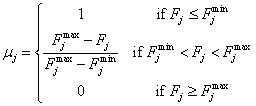(33)

where μj is membership value of objective j, and Fj max and Fj min are maximum and minimum values of objective j, respectively.

For each non-dominated solution, the membership function is normalized as follows :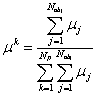(34)

where μk is membership function of non-dominated solution k; Nobj is the number of objective functions; and NP is the number of Paretooptimal solutions.

The solution with maximum membership function μk can be chosen as the best compromise solution for the problem.

#### Numerical Results

The proposed PG-PSOCF has been tested on the IEEE 30-bus with two objectives including total operation cost and emission. The test system has 41 transmission lines, six generators at buses 2, 5, 8, 11, and 13, and four transformers at lines 6-9, 6-10, 4-12 and 27-28. The total load demand of the system is 283.4 MW and 126.2 MVar. The data for the system can be found in [1,33]. The data for total cost, emission and transmission line limits is given in Table 1 and power flow limits of transmission lines are given in Table 2.

G1
(bus 1)
G2
(bus 2)
G3
(bus 5)
G4
(bus 8)
G5
(bus 11)
G6
(bus 13)
Cost coefficients
ai (\$/h) 0 0 0 0 0 0
bi (\$/MWh) 2 1.75 1 3.25 3 3
ci (\$/MW2h) 0.00375 0.0175 0.0625 0.00834 0.025 0.025
Emission coefficients
αi (ton/h) 0.04091 0.02543 0.04258 0.05326 0.04258 0.06131
βi (ton/MWh) -20.05554 -0.06047 -0.05094 -0.03550 -0.05094 -0.05555
γi (ton/MW2h) 0.06490 0.05638 0.04586 0.03380 0.04586 0.05151
ξi (ton/h) 0.0002 0.0005 0.000001 0.002 0.000001 0.00001
λi (1/MW) 2.857 3.333 8.000 2.000 8.000 6.667

Table 1: Cost and emission coefficients for generators.

 Line 1-2 1-3 2-4 3-4 2-5 2-6 4-6 5-7 6-7 6-8 6-9 Pl,max MW) 130 130 65 130 130 65 90 70 130 32 65 Line 6-10 9-10 9-11 4-12 12-13 12-14 12-15 12-16 14-15 15-18 16-17 Pl,max MW) 32 65 65 65 65 32 32 32 16 16 16 Line 18-19 19-20 10-20 10-17 10-21 10-22 21-22 15-23 22-24 23-24 24-25 Pl,max MW) 16 32 32 32 32 32 32 16 16 16 16 Line 25-26 25-27 28-27 27-29 27-30 29-30 8-28 6-28 Pl,max MW) 16 16 65 16 16 16 32 32

Table 2: Limits of transmission lines.

For obtaining the power flow solution of the system, the Matpower toolbox  is used. Since the bus voltage limits have a great effect on the final results. Therefore, in this research two kinds of bus voltage limit at buses are considered in the range [0.95, 1.05] and [0.95, 1.10]. The tap changer limit of transformers is set to [0.9, 1.1] for all cases. The two capacitor banks are installed at buses 10 and 24.

The proposed PG-PSOCF is coded in the Matlab platform and run on a 3.2 GHz PC. The control parameters of the proposed PG-PSOCF method for all cases of the test system are simply selected as follows: NP=10, c1=c2=2.05, R=0.15, Itmax=200. For each test case, the proposed method is performed 20 independent runs.

Cost objective function

In this case, there is only the total cost objective function is considered. The results obtained by the PG-PSOCF method including min total cost, average total cost, max total cost, standard deviation and average computational time for two kinds of bus voltage limits are given in Table 3. As observed from the table, the total cost for the case with bus voltage limit of 1.05 pu is higher than that for the case with bus voltage limit of 1.1 pu while the total emission for the two cases are nearly the same. For the both cases, the standard deviation is very small which indicates that the proposed method can obtain high quality solution for this case.

Vbusmax = 1.05 pu Vbusmax = 1.10 pu
Min total cost (\$/h) 802.2801 799.1994
Average total cost (\$/h) 802.7527 799.9818
Max total cost (\$/h) 805.4520 804.4023
Standard deviation (\$/h) 0.9124 1.2758
Average CPU time (s) 15.335 15.248
Total emission (ton/h)     0.3631 0.3666
Power losses (MW) 9.4364 8.6699

Table 3: Results by PG-PSOCF for cost dispatch case with different bus voltage limits.

The best results by the proposed PG-PSOCF for the two cases have been compared to those from other methods as shown in Tables 4 and 5. For the both cases, the proposed method can obtain better total cost than the others. Therefore, the proposed PG-PSO is very effective for solving the OPF problem.

NLP  EP  TS  IEP  MDE-OPF  MSLFA  PG-PSOCF
Pg1 (MW) 176.26 173.848 176.04 176.2358 175.974 179.1929 176.0340
Pg2 (MW) 48.84 49.998 48.76 49.0093 48.884 48.9804 48.8786
Pg3 (MW) 21.51 21.386 21.56 21.5023 21.51 20.4517 21.5350
Pg4 (MW) 22.15 22.63 22.05 21.8115 22.24 20.9264 22.1439
Pg5 (MW) 12.14 12.928 12.44 12.3387 12.251 11.5897 12.2448
Pg6 (MW) 12 12 12 12.0129 12 11.9579 12.0000
Cost (\$/h) 802.4 802.62 802.29 802.465 802.376 802.287 802.2801

Table 4: Result comparison for cost dispatch case with bus voltage limit of 1.05 pu.

PSO  IPSO  PG-PSOCF
Pg1 (MW) 178.4646 177.0431 177.2254
Pg2 (MW) 46.274 49.209 48.6302
Pg3 (MW) 21.4596 21.5135 21.3220
Pg4 (MW) 21.446 22.648 21.0422
Pg5 (MW) 13.207 10.4146 11.8500
Pg6 (MW) 12.0134 12 12.0000
Cost (\$/h) 802.205 801.978 799.1994

Table 5: Result comparison for cost dispatch case with bus voltage limit of 1.1 pu.

Emission objective function

In this case, there is only the emission objective function is considered. The obtained results by the proposed method for the two cases of bus voltage limits including min emission, average emission, max emission, standard deviation, and average CPU time are given in Table 6. The total emission for the both cases of bus voltage limits is not different. Moreover, the standard deviation of the proposed method for the both cases is also very small.

Vbusmax = 1.05 pu Vbusmax = 1.10 pu
Min emission (ton/h) 0.2049 0.2048
Average emission (ton/h) 0.2092 0.2063
Max emission (ton/h) 0.2398 0.2195
Standard deviation (\$/h) 0.0087 0.0032
Average CPU time (s) 15.137 15.241
Total cost (\$/h)     944.7824 943.7578
Power losses (MW) 3.3514 3.0357

Table 6: Results by PG-PSOCF for emission dispatch case with different bus voltage limits.

The result comparisons from the proposed method and other methods for this case with two bus voltage limits are given in Tables 7 and 8. As shown in the tables, the total emission from the proposed method is less than that from the others. Therefore, the proposed PGPSOCF is also very effective for this case.

GA  PSO  SLFA  MSLFA  PG-PSOCF
Pg1 (MW) 78.2885 59.8075 64.4840 65.7798 63.9471
Pg2 (MW) 68.1602 80 71.3870 68.2688 67.4886
Pg3 (MW) 46.7848 50 49.8573 50 50.0000
Pg4 (MW) 33.4909 35 35 34.9999 35.0000
Pg5 (MW) 30 27.1398 30 29.9982 30.0000
Pg6 (MW) 36.3713 40 39.9729 39.9970 40.0000
Emission (ton/h) 0.21170 2.096 2.063 0.2056 0.2049

Table 7: Best result comparison for emission dispatch case with bus voltage limit of 1.05 pu.

GA  PSO  IPSO  PG-PSOCF
Pg1 (MW) 69.7300 67.1300 67.0400 63.9471
Pg2 (MW) 67.8400 68.9400 68.1400 67.4886
Pg3 (MW) 49.7300 49.8600 50.0000 50.0000
Pg4 (MW) 34.4200 34.8900 35.0000 35.0000
Pg5 (MW) 29.1500 29.6700 30.0000 30.0000
Pg6 (MW) 39.2900 39.9400 40.0000 40.0000
Emission (ton/h) 0.2072 0.2063 0.2058 2.048

Table 8: Best result comparison for emission dispatch case with bus voltage limit of 1.1 pu.

Multiobjective function

In this case, both total cost and emission are simultaneously considered in the problem. Since there is not much different total cost and emission between the bus voltage limits, only the case with bus voltage limit of 1.05 pu is considered for the multiobjective function. For obtaining the Pareto front for this case, multiple solutions are determined by changing the value of weight factor ω from 0 to 1. Figure 1 depicts the Pareto front obtained by the proposed method for different bus voltage limits.

Based on the obtained solution for the Pareto front, the fuzzy based mechanism is used for obtaining the best compromise solution for the problem. The best compromise solution obtained by the proposed method is 866.0267 (\$/h) and 0.2229 (ton/h) which is better than other methods as shown in Table 9. Therefore, the proposed PG-PSOCF is also very effective for the multiobjective case of the problem.

GA  PSO  SLFA  MSLFA  PG-PSOCF
Pg1 (MW) 96.1251 97.8588 98.9772 97.55027 95.0194
Pg2 (MW) 68.5168 61.9419 58.6832 60.42367 61.4059
Pg3 (MW) 26.7031 31.1310 35.0661 31.6343 31.9402
Pg4 (MW) 35 34.4808 31.7585 35 35.0000
Pg5 (MW) 30 29.7100 29.9182 30 30.0000
Pg6 (MW) 34.7555 36.0884 35.8174 35.21483 35.1872
Total cost (\$/h) 872.9601 872.8731 872.8533 867.713 866.0267
Emission (ton/h) 0.2270 0.2253 0.2249 0.2247 0.2229

Table 9: Best result comparison for multiobjective dispatch case with bus voltage limit of 1.05 pu.

#### Conclusion

In this paper, the proposed PG-PSOCF method has been effectively and efficiently implemented for solving the MOOPF problem. The PGPSOCF is the conventional PSO method with constriction factor guided by pseudo-gradient for enhancement its search ability and solution quality. The proposed can properly deal with the MOOPF problem using the fuzzy based mechanism for best compromise solution. The test results for the IEEE 30 bus system with different bus voltage limits have indicated that the proposed method can obtain better solution quality than many other methods. Moreover, the proposed method can be also extended for dealing with more complex and larger scale OPF problems. Therefore, the proposed PG-PSOCF could be a powerful and favorable method for solving the MOOPF problem.

#### Acknowledgement

This research is funded by Vietnam National University HoChiMinh City (VNUHCM) under grant number C2014-20-24.

#### References

Select your language of interest to view the total content in your interested language

### Article Usage

• Total views: 13057
• [From(publication date):
December-2015 - Sep 17, 2019]
• Breakdown by view type
• HTML page views : 9050

## Post your commentCan't read the image? click here to refresh
###### Peer Reviewed Journals

Make the best use of Scientific Research and information from our 700 + peer reviewed, Open Access Journals

International Conferences 2019-20

Meet Inspiring Speakers and Experts at our 3000+ Global Annual Meetings

Top